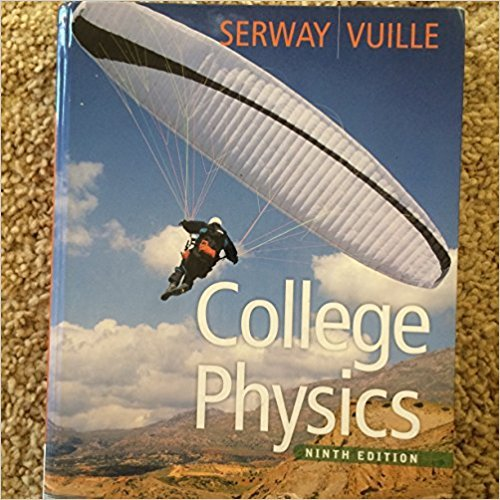×
Log in to StudySoup
Get Full Access to College Physics - 9 Edition - Chapter 2 - Problem 2.4
Join StudySoup for FREE
Get Full Access to College Physics - 9 Edition - Chapter 2 - Problem 2.4

Already have an account? Login here
×
Reset your password

# Solved: A juggler throws a bowling pin straight up in theISBN: 9780840062062 220

## Solution for problem 2.4 Chapter 2

College Physics | 9th Edition

• Textbook Solutions
• 2901 Step-by-step solutions solved by professors and subject experts
• Get 24/7 help from StudySoup virtual teaching assistantsCollege Physics | 9th Edition

4 5 1 344 Reviews
15
3
Problem 2.4

A juggler throws a bowling pin straight up in the air. After the pin leaves his hand and while it is in the air, which statement is true? (a) The velocity of the pin is always in the same direction as its acceleration. (b) The velocity of the pin is never in the same direction as its acceleration. (c) The acceleration of the pin is zero. (d) The velocity of the pin is opposite its acceleration on the way up. (e) The velocity of the pin is in the same direction as its acceleration on the way up.

Step-by-Step Solution:
Step 1 of 3

Chapter 8 Kinetic Energy- describes the motion of the object. It‘s given by the following formula: 1 2 K= mv 2 The SI unit for Kinetic Energy is a Joule which is equal to a N*m Potential Energy is another form of energy. It is given by: PE=mgh To find gravitational potential energy: m1m 2 U G( )G r To find the potential energy stored in a spring we use a formula to find the elastic potential energy: 1 2

Step 2 of 3

Step 3 of 3

##### ISBN: 9780840062062

This full solution covers the following key subjects: . This expansive textbook survival guide covers 30 chapters, and 2181 solutions. College Physics was written by and is associated to the ISBN: 9780840062062. Since the solution to 2.4 from 2 chapter was answered, more than 269 students have viewed the full step-by-step answer. This textbook survival guide was created for the textbook: College Physics, edition: 9. The full step-by-step solution to problem: 2.4 from chapter: 2 was answered by , our top Physics solution expert on 01/05/18, 06:15PM. The answer to “A juggler throws a bowling pin straight up in the air. After the pin leaves his hand and while it is in the air, which statement is true? (a) The velocity of the pin is always in the same direction as its acceleration. (b) The velocity of the pin is never in the same direction as its acceleration. (c) The acceleration of the pin is zero. (d) The velocity of the pin is opposite its acceleration on the way up. (e) The velocity of the pin is in the same direction as its acceleration on the way up.” is broken down into a number of easy to follow steps, and 98 words.

Unlock Textbook Solution

Enter your email below to unlock your verified solution to:

Solved: A juggler throws a bowling pin straight up in the